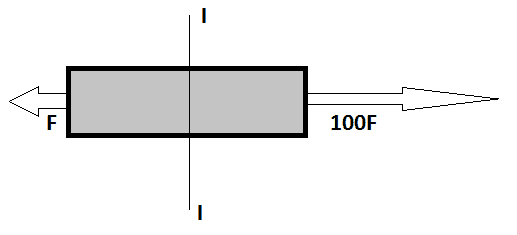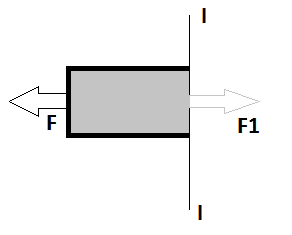# What will be stress in the unbalanced force situation

Ravi Singh choudhary
< Mentor Note -- thread moved to HH from the technical physics forums, so no HH Template is shown >Problem Statement:
Case 1: Mass is m; Cross sectional area is uniform A. What will be the stress in the middle section.
Case 2: Bar is massless; What will be the stress in the middle section.
Case 3: Bar is still assumed massless but 100F is increased to 500F; What will be the stress in the middle section.

Relevant equation: F/A; point is to find internal force in each situation.

My attempt to the solution: I found the acceleration simply by dividing "m" to the net force "99F". My poor understanding about stress was; it only acts when body in is zero unbalanced force. Simply we have to find the internal force at point which stress need to be calculated. But I realized even after there is unbalanced force situation we have internal force at particular section.

So I solved for case I: a=(99F/m)
After solving equation F1-F=(m/2)*a; I found the value of F1 i.e. (101/2)F
stress will be F1/A.But solution given was 99F/A i.e. stress due to original force configuration at middle section.

For Case 2 and Case 3; I am clueless.

Last edited by a moderator:

Gold Member
Think about the forces acting on the individual wagons in a train which is being accelerated by the pull of an engine .

Mentor
< Mentor Note -- thread moved to HH from the technical physics forums, so no HH Template is shown >

View attachment 101475
Problem Statement:
Case 1: Mass is m; Cross sectional area is uniform A. What will be the stress in the middle section.
Case 2: Bar is massless; What will be the stress in the middle section.
Case 3: Bar is still assumed massless but 100F is increased to 500F; What will be the stress in the middle section.

Relevant equation: F/A; point is to find internal force in each situation.

My attempt to the solution: I found the acceleration simply by dividing "m" to the net force "99F". My poor understanding about stress was; it only acts when body in is zero unbalanced force. Simply we have to find the internal force at point which stress need to be calculated. But I realized even after there is unbalanced force situation we have internal force at particular section.

So I solved for case I: a=(99F/m)
After solving equation F1-F=(m/2)*a; I found the value of F1 i.e. (101/2)F
stress will be F1/A.
View attachment 101476
I confirm this answer. Nicely done.
But solution given was 99F/A i.e. stress due to original force configuration at middle section.
In my judgment, this is not correct.
For Case 2 and Case 3; I am clueless.
In case 1, the mass of the bar is not specified. So, we can make the mass as small as we wish (of course, the acceleration will get higher and higher). However, in the limit of very small masses, the answer for case 2 will be the same as for case 1. In case 3, you apply the same methodology as for cases 1 and 2.

•Ravi Singh choudhary